Math - 2015 Past Year Paper

# Math - 2015 Past Year Paper - IIT JAM

Test Description

## 60 Questions MCQ Test IIT JAM Past Year Papers and Model Test Paper (All Branches) - Math - 2015 Past Year Paper

Math - 2015 Past Year Paper for IIT JAM 2023 is part of IIT JAM Past Year Papers and Model Test Paper (All Branches) preparation. The Math - 2015 Past Year Paper questions and answers have been prepared according to the IIT JAM exam syllabus.The Math - 2015 Past Year Paper MCQs are made for IIT JAM 2023 Exam. Find important definitions, questions, notes, meanings, examples, exercises, MCQs and online tests for Math - 2015 Past Year Paper below.
Solutions of Math - 2015 Past Year Paper questions in English are available as part of our IIT JAM Past Year Papers and Model Test Paper (All Branches) for IIT JAM & Math - 2015 Past Year Paper solutions in Hindi for IIT JAM Past Year Papers and Model Test Paper (All Branches) course. Download more important topics, notes, lectures and mock test series for IIT JAM Exam by signing up for free. Attempt Math - 2015 Past Year Paper | 60 questions in 180 minutes | Mock test for IIT JAM preparation | Free important questions MCQ to study IIT JAM Past Year Papers and Model Test Paper (All Branches) for IIT JAM Exam | Download free PDF with solutions
 1 Crore+ students have signed up on EduRev. Have you?
Math - 2015 Past Year Paper - Question 1

### Suppose N is a normal subgroup of a group G. Which one of the following is true?

Math - 2015 Past Year Paper - Question 2

### Let y(x) = u(x) sin sin x + v(x) cos x be a solution of the differential equation y” + y = sec x. Then u(x) is

Math - 2015 Past Year Paper - Question 3

### Let a, b, c, d be distinct non- zero real numbers with a + b = c + d. Then an eigenvalue of the matrix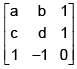is

Math - 2015 Past Year Paper - Question 4

Let S be a nonempty subset of R. If S is a finite union of disjoint bounded intervals, then which one of the following is true?

Math - 2015 Past Year Paper - Question 5

Let {xn} be a convergent sequence of real numbers.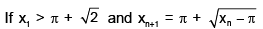for n ≥ 1, then which one of the following is the limit of this sequence?

Math - 2015 Past Year Paper - Question 6

The volume of the portion of the solid cylinder x2 + y2 ≤ 2 bounded above by the surface z = x2 + y2 and bounded below by the xy- plane is

Math - 2015 Past Year Paper - Question 7

Let f: R→R be a differentiable function with f(0) = 0. If for all x ∈ R, 1 < f'(x) < 2, then which one of the following statements is true on (0, ∝)?

Math - 2015 Past Year Paper - Question 8

If an integral curve of the differential equation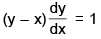passes through (0, 0) and (α, 1),
then α is equal to

Math - 2015 Past Year Paper - Question 9

An integrating factor of the differential equation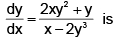Math - 2015 Past Year Paper - Question 10

Let A be a nonempty subset of R Let I(A) denote the set of interior points of A. Then I(A) can be

Math - 2015 Past Year Paper - Question 11

Let S3 be the group of permutations of three distinct symbols. The direct sum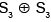has an element of order

Math - 2015 Past Year Paper - Question 12

The orthogonal trajectories of the family of curves y = C1x3 are

Math - 2015 Past Year Paper - Question 13

Let G be a nonabelian group. Let α ∈ G have order 4 and let β ∈ G have order 3. Then the order of the element αβ in G

Math - 2015 Past Year Paper - Question 14

Let S be the bounded surface of the cylinder x2 + y2 = 1 cut by the planes z = 0 and z = 1 + x. Then the value of the surface integral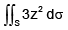is equal to

Math - 2015 Past Year Paper - Question 15

Suppose that the dependent variables z and w are functions of the independent variables x and y, defined by the equations f(x, y, z, w) = 0 and g(x, y, z, w) = 0, where fzgw – fwgz = 1.
Which one of the following is correct

Math - 2015 Past Year Paper - Question 16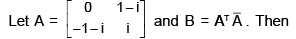Math - 2015 Past Year Paper - Question 17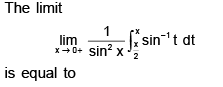Math - 2015 Past Year Paper - Question 18

Let P2(R) be the vector space of polynomials in x of degree at most 2 with real coefficients. Let M2(R) be the vector space of 2 × 2 real matrices. If a linear transformation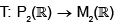is defined as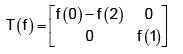then

Math - 2015 Past Year Paper - Question 19

Let B1 = {(1, 2), (2, –1)} and B2 = {(1, 0), (0, 1} be ordered bases of R2 . If T : R2 →  R2  is a linear transformation such that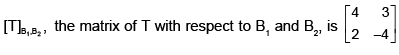then T(5, 5) is equal to

Math - 2015 Past Year Paper - Question 20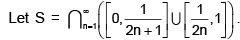Which one of the following statements is FALSE?

Math - 2015 Past Year Paper - Question 21

Let f : R → R be a strictly increasing continuous function. If {an} is a sequence in [0, 1], then the sequence {f(an)} is

Math - 2015 Past Year Paper - Question 22

Which one of the following statements is true for the series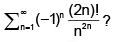Math - 2015 Past Year Paper - Question 23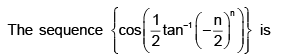Math - 2015 Past Year Paper - Question 24

If y(t) is a solution of the differential equation y” + 4y = 2et, then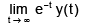is equal to

Math - 2015 Past Year Paper - Question 25

For what real values of x and y, does the integral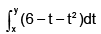attain its maximum?

Math - 2015 Past Year Paper - Question 26

The area of the planar region bounded by the curves x = 6y2 – 2 and x = 2y2 is

Math - 2015 Past Year Paper - Question 27

For n ≥ 2, let fn: R → R be given by fn(x) = xn sin x. Then at x = 0, fn has a

Math - 2015 Past Year Paper - Question 28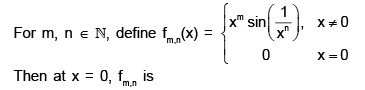Math - 2015 Past Year Paper - Question 29

Let G and H be nonempty subsets of R , where G is connected and G U H is not connected.
Which one of the following statements is true for all such G and H ?

Math - 2015 Past Year Paper - Question 30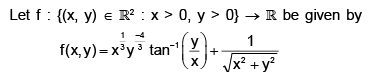Then the value of

*Multiple options can be correct
Math - 2015 Past Year Paper - Question 31

Let f: R → R be a function defined by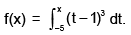In which of the following interval(s), f takes the value 1?

*Multiple options can be correct
Math - 2015 Past Year Paper - Question 32

Which of the following statements is (are) true?

*Multiple options can be correct
Math - 2015 Past Year Paper - Question 33

Which of the following conditions implies (imply) the convergence of a sequence {xn} of real numbers?

*Multiple options can be correct
Math - 2015 Past Year Paper - Question 34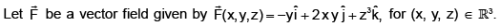If C is the curve of intersection of the surfaces x2 + y2 = 1 and y + z = 2, then which of the following is (are) equal to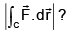*Multiple options can be correct
Math - 2015 Past Year Paper - Question 35

Let V be the set of 2 × 2 matrices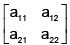with complex entries such that a11 + a22 = 0. Let W be the set of matrices in V with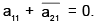. Then, under usual matrix addition and scalar multiplication, which of the following is (are) true?

*Multiple options can be correct
Math - 2015 Past Year Paper - Question 36

The initial value problem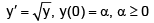has

*Multiple options can be correct
Math - 2015 Past Year Paper - Question 37

Which of the following statements is (are) true on the interval (0, π/2) ?

*Multiple options can be correct
Math - 2015 Past Year Paper - Question 38

Let f: R2 → R be defined by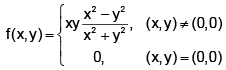At (0,0)

*Multiple options can be correct
Math - 2015 Past Year Paper - Question 39

Let f, g : [0, 1] → [0, 1] be functions. Let R(f) and R(g) be the ranges of f and g, respectively.
Which of the following statements is (are) true?

*Multiple options can be correct
Math - 2015 Past Year Paper - Question 40

Let f: (–1, 1) → R be the function defined by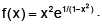Then

*Answer can only contain numeric values
Math - 2015 Past Year Paper - Question 41

Let C be the straight line segment from P(0, π) to (4, π/2) in the xy- plane. Then the value of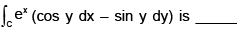*Answer can only contain numeric values
Math - 2015 Past Year Paper - Question 42

Let S be the portion of the surface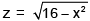bounded by the planes x = 0, x = 2, y = 0, and y = 3. The surface area of S, correct upto three decimal places, is ____________

*Answer can only contain numeric values
Math - 2015 Past Year Paper - Question 43

The number of distinct normal subgroups of S3 is _____

*Answer can only contain numeric values
Math - 2015 Past Year Paper - Question 44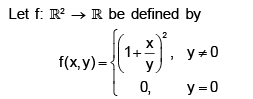If the directional derivative of f at (0, 0) exists along the direction cos αi sin α j , where sin a ≠  0, then the value of cot a is _________

*Answer can only contain numeric values
Math - 2015 Past Year Paper - Question 45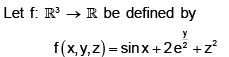The maximum rate of change of f at (π/4 , 0, 1) correct upto three decimal places, is ________

*Answer can only contain numeric values
Math - 2015 Past Year Paper - Question 46

If the power series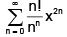converges for |x| < c and diverges for |x| > c, then the value of c, correct upto three decimal places, is--

*Answer can only contain numeric values
Math - 2015 Past Year Paper - Question 47

If 52015 ≡  n. ( mod 11) and n ∈ {0, 1, 2, 3, 4, 5, 6, 7, 8, 9, 10}, then n is equal to _________

*Answer can only contain numeric values
Math - 2015 Past Year Paper - Question 48

If the set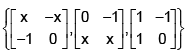is linearly dependent in the vector space of all 2 × 2 matrices with real entries, then x is equal to ___

*Answer can only contain numeric values
Math - 2015 Past Year Paper - Question 49

let f: R → R be defined by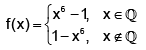The number of points at which f is continuous, is ______________

*Answer can only contain numeric values
Math - 2015 Past Year Paper - Question 50

Let f: (0, 1) → R be a continuously differentiable function such that f' has finitely many zeros in (0, 1) and fchanges sign at exactly two of these points. Then for any y ∈ R , the maximum number of solutions to f(x) = y in (0, 1) is ______________

*Answer can only contain numeric values
Math - 2015 Past Year Paper - Question 51

Let R be the planar region bounded by the lines x = 0, y = 0 and the curve x2 + y2 = 4, in the first quadrant. Let C be the boundary of R, oriented counter- clockwise. Then the value of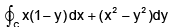is ----

*Answer can only contain numeric values
Math - 2015 Past Year Paper - Question 52

Suppose G is a cyclic group and σ, τ ∈ G are such that order(σ) = 12 and order (τ) = 21. Then the order of the smallest group containing σ and τ is ______________

*Answer can only contain numeric values
Math - 2015 Past Year Paper - Question 53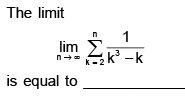*Answer can only contain numeric values
Math - 2015 Past Year Paper - Question 54

Let M2(R) be the vector space of 2 × 2 real matrices. Let V be a subspace of M2(R) defined by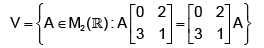Then the dimension  is ____

*Answer can only contain numeric values
Math - 2015 Past Year Paper - Question 55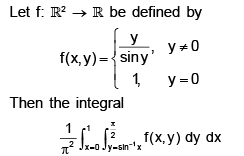correct upto three decimal places, is _________

Detailed Solution for Math - 2015 Past Year Paper - Question 55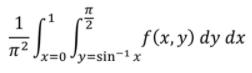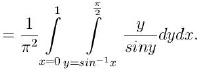We now change the order of integration. Then the integral equals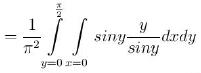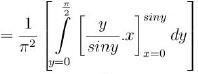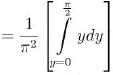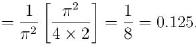*Answer can only contain numeric values
Math - 2015 Past Year Paper - Question 56

The coefficient of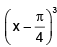in the Taylor series expansion of the function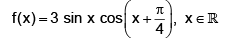about the point  π/4 correct upto three decimal places, is ______

*Answer can only contain numeric values
Math - 2015 Past Year Paper - Question 57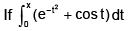as the power series expansion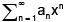then a5  correct upto three decimal places, is equal to _

Detailed Solution for Math - 2015 Past Year Paper - Question 57

We have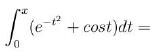a1x + a2x2 + a3x3 + a4x4 + a5x5 +..
On differentiation, it gives, by Leibnitz rule on the left, and termwise differentiation on the right,
ex = 1 +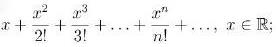cosx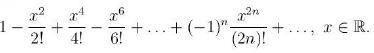Then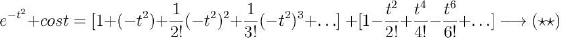The coefficient of t4 in (*) is 5a5.

The coefficient of x4 in (**) is 1/2 + 1/4!, i.e., 1/2 + 1/24 = 13/24.

Comparing these coefficients (note that symbols x and t play the same role), we get
13/24 = 5a5
Which given a5 = 1/5. 13/24 ≈ 0.108.

*Answer can only contain numeric values
Math - 2015 Past Year Paper - Question 58

Let ℓ be the length of the portion of the curve x = x(y) between the lines y = 1 and y = 3, where x(y) satisfies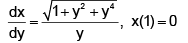The value of ℓ, correct upto three decimal places, is ___________

*Answer can only contain numeric values
Math - 2015 Past Year Paper - Question 59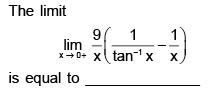*Answer can only contain numeric values
Math - 2015 Past Year Paper - Question 60

Let P and Q be two real matrices of size 4 × 6 and 5 × 4, respectively. If rank(Q) = 4 and rank(QP) = 2, then rank(P) is equal to ______

## IIT JAM Past Year Papers and Model Test Paper (All Branches)

8 docs|48 tests
Information about Math - 2015 Past Year Paper Page
In this test you can find the Exam questions for Math - 2015 Past Year Paper solved & explained in the simplest way possible. Besides giving Questions and answers for Math - 2015 Past Year Paper, EduRev gives you an ample number of Online tests for practice

## IIT JAM Past Year Papers and Model Test Paper (All Branches)

8 docs|48 tests(Scan QR code)